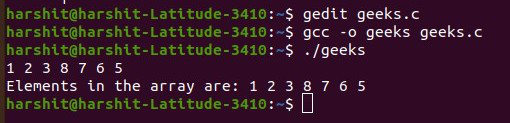# C program to input an array from a sequence of space-separated integers

• Last Updated : 15 Jul, 2021

Given a string S consisting of space-separated integers, the task is to write a C program to take the integers as input from the string S and store them in an array arr[].

Examples:

Input: S = “1 2 3 4”
Output: {1, 2, 3, 4}

Input: S = “32 12”
Output: {32, 12}

Approach: The idea is to solve the given problem is to use getchar() function to check if a ‘\n’ (newline) occurs is found while taking input and then stop the input. Follow the step below to solve the given problem:

• Initialize a variable, say count, which is used to store the index of the array element.
• Initialize an array arr[] of size 106 to store the elements into the array.
• Iterate using a do-while loop until newLine occurs and perform the following steps:
• Store the current value at index count as scanf(“%d “, &arr[count]); and increment the value of count.
• If the next character is not endline, then continue. Otherwise, break out of the loop.
• After completing the above steps, print the elements stored in the array.

Below is the implementation of the above approach:

## C

 `// C program for the above approach` `#include `   `// Driver Code` `int` `main()` `{` `    ``// Stores the index where the` `    ``// element is to be inserted` `    ``int` `count = 0;`   `    ``// Initialize an array` `    ``int` `a;`   `    ``// Perform a do-while loop` `    ``do` `{`   `        ``// Take input at position count` `        ``// and increment count` `        ``scanf``(``"%d"``, &a[count++]);`   `        ``// If '\n' (newline) has occurred` `        ``// or the whole array is filled,` `        ``// then exit the loop`   `        ``// Otherwise, continue` `    ``} ``while` `(``getchar``() != ``'\n'` `&& count < 100);`   `    ``// Resize the array size to count` `    ``a[count];`   `    ``// Print the array elements` `    ``for` `(``int` `i = 0; i < count; i++) {` `        ``printf``(``"%d, "``, a[i]);` `    ``}`   `    ``return` `0;` `}`

Output:My Personal Notes arrow_drop_up
Recommended Articles
Page :